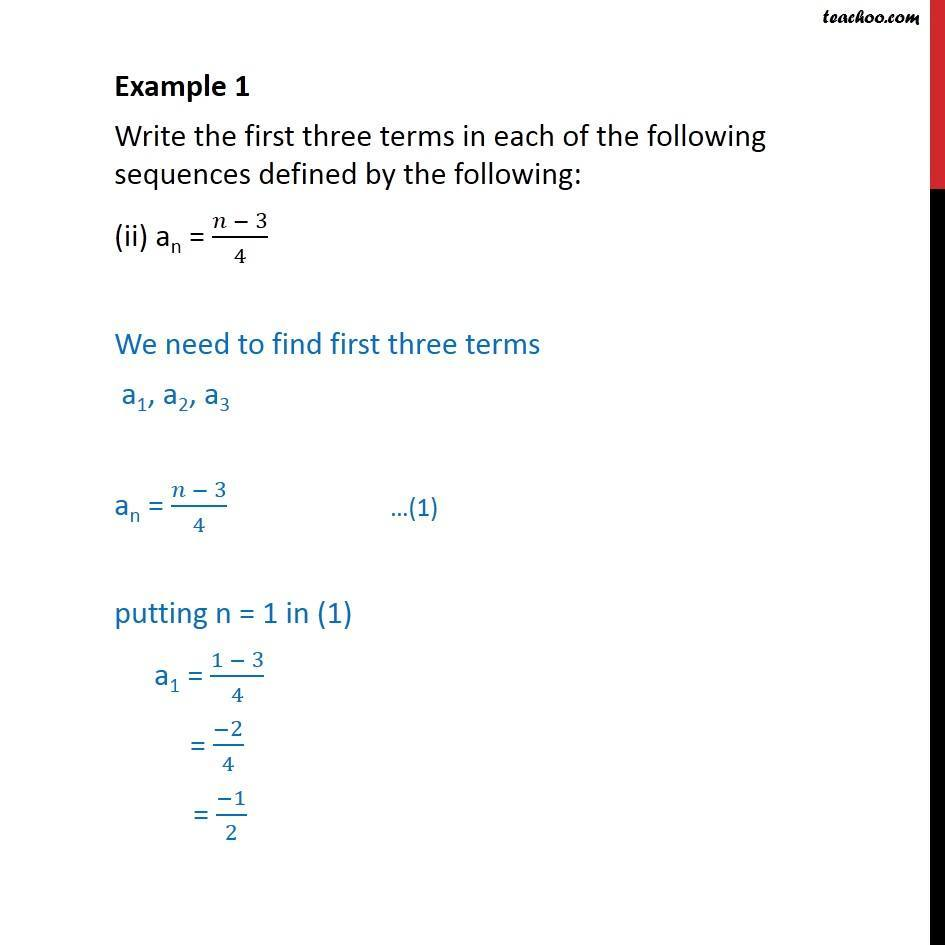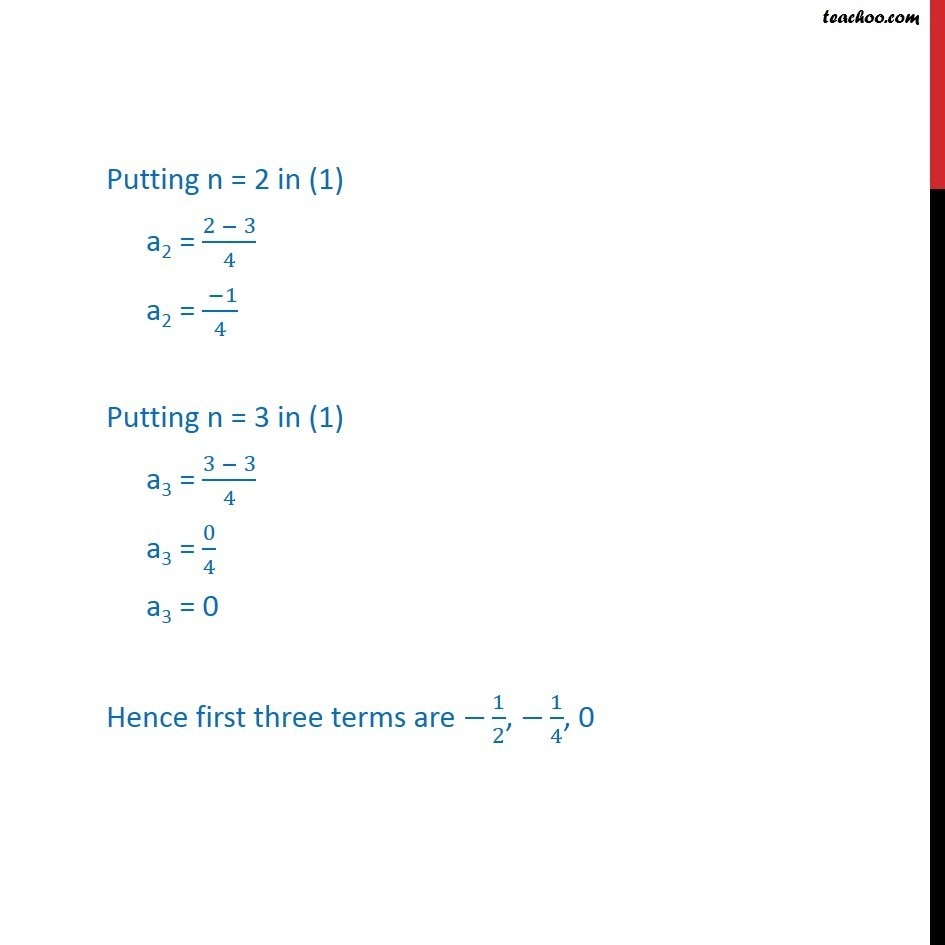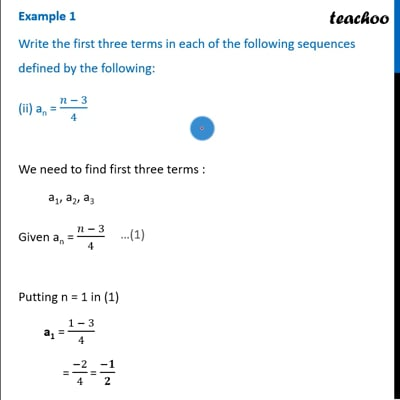Examples

Chapter 8 Class 11 Sequences and Series
Serial order wiseThis video is only available for Teachoo black usersThis video is only available for Teachoo black users

Learn in your speed, with individual attention - Teachoo Maths 1-on-1 Class

### Transcript

Example 1 Write the first three terms in each of the following sequences defined by the following: (ii) an = (𝑛 − 3)/4 We need to find first three terms a1, a2, a3 an = (𝑛 − 3)/4 putting n = 1 in (1) a1 = (1 − 3)/4 = (−2)/4 = (−1)/2 Putting n = 2 in (1) a2 = (2 − 3)/4 a2 = ( −1)/4 Putting n = 3 in (1) a3 = (3 − 3)/4 a3 = 0/4 a3 = 0 Hence first three terms are −1/2, −1/4, 0